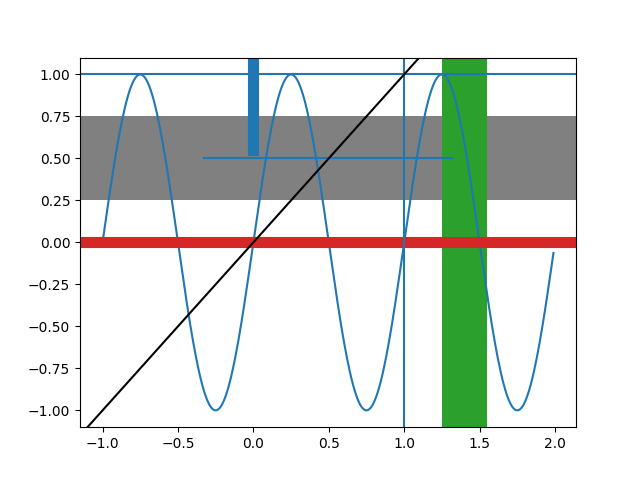# axhspan Demo#

Create lines or rectangles that span the axes in either the horizontal or vertical direction, and lines than span the axes with an arbitrary orientation.import numpy as np
import matplotlib.pyplot as plt

t = np.arange(-1, 2, .01)
s = np.sin(2 * np.pi * t)

fig, ax = plt.subplots()

ax.plot(t, s)
# Thick red horizontal line at y=0 that spans the xrange.
ax.axhline(linewidth=8, color='#d62728')
# Horizontal line at y=1 that spans the xrange.
ax.axhline(y=1)
# Vertical line at x=1 that spans the yrange.
ax.axvline(x=1)
# Thick blue vertical line at x=0 that spans the upper quadrant of the yrange.
ax.axvline(x=0, ymin=0.75, linewidth=8, color='#1f77b4')
# Default hline at y=.5 that spans the middle half of the axes.
ax.axhline(y=.5, xmin=0.25, xmax=0.75)
# Infinite black line going through (0, 0) to (1, 1).
ax.axline((0, 0), (1, 1), color='k')
# 50%-gray rectangle spanning the axes' width from y=0.25 to y=0.75.
ax.axhspan(0.25, 0.75, facecolor='0.5')
# Green rectangle spanning the axes' height from x=1.25 to x=1.55.
ax.axvspan(1.25, 1.55, facecolor='#2ca02c')

plt.show()


Gallery generated by Sphinx-Gallery